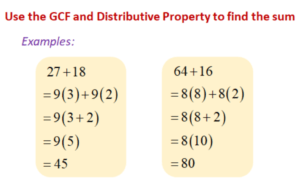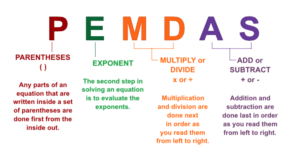# MS Math – Oct 4-8, 2021

1.6th Grade Math – Mrs. Evans

This week we will finish Chapter 1 with a test on Wednesday.   We will carry with us the ability to do all decimal arithmetic!  For help with decimal arithmetic, see the link to the Math Antics video in last week’s post.   Also in this chapter we learned about finding the least common multiple (LCM) and greatest common factor (GCF) and how to use these values to solve real world problems.  One of the important properties that we have reviewed in this chapter is the distributive property.  This is a property that we will use a great deal in algebra.  The distributive property allows us to distribute a factor across a sum.  Here is how it looks:After we finish with the Chapter 1 test, we will continue to learn more about decimals in Chapter 2.

Math Grade Math – Mrs. Evans and Mrs. VonFeldt

This week we will review and test on Module 2 which covers multiplying and dividing integers.  If we remember the rules (shown in last week’s post), it can be a straight forward process.  It is, however, necessary that we continue to ALWAYS use the proper order of operations.  Here is a reminder of PEMDAS which helps us keep  the order correct:For students who need help with Module 2 concepts, here is a video summarizing how we multiply and divide integers.

When we finish with the Module 2 test, we will begin to apply what we have learned about negatives to rational numbers (fractions, decimals and mixed numbers).

8th Grade Math – Mrs. Ernest

This week students will begin solving multi-step algebraic equations. Students will be working through the remainder of Chapter 1, and we will begin Chapter 2 by the end of this week. I will be specifically looking for each student to write out their work as clearly as possible! See the image below for an example one-step problem and the requirements for showing work on homework.Algebra – Mrs. VonFeldt

This week is all about solving linear equations. By the end of the week, students should be able to solve all types of one variable equations using the following steps:

• Distribute;
• Combine like terms;